AnyLogic

# Arrays

Some problems require multi-dimensional data. Alike some other traditional system dynamics tools, AnyLogic supports arrays. An array is a storage of numbers that may have any number of dimensions. Each dimension has finite number of indices — subscripts.

Arrays are used when it is necessary to store a large set of coefficients and access them or when there are multiple model layers. The latter case is useful when you have defined a model for some subsystem and there are other subsystems, which have the same structure, as the first one, but other numerical parameters. One can implement such multi-dimensional models making copies of the default diagram and changing the parameters. Such approach has one great disadvantage: if you want to change the model, you need do it so much times, as many layers you have; the diagram grows and becomes incomprehensible. Array allows you to create a single diagram for all the layers. Therefore, model remains compact, and changes you make will affect the whole model, but not a single layer.

For example, you create a model of a nation's health, describing some social or health processes in respect to different groups of population. You may need to separate people by three characteristics: gender, age group, and social group. This example fits well in the array concept. Instead of dealing with multiple models describing different groups of people, you can just define an array with such enumerations: Gender(male, female), Age(child, teenager, adult, aged), and SocialGroup(wealthy, middleclass, deprived).

The following variables can be made arrays in AnyLogic: stock, flow, dynamic variable, parameter.

## Defining an array variable

To define an array variable

1. Select the variable in a graphical editor or in the Projects view.
2. To make the selected variable a variable of type array, check the Array check box in the Properties view.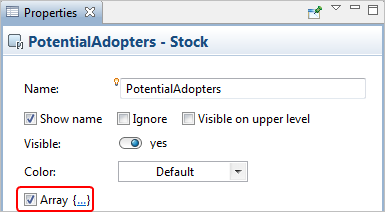3. You can see that the variable’s name in the graphical editor is now followed with square brackets. In this way AnyLogic visualizes array variables.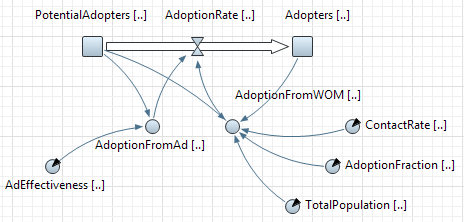Array variables in the graphical editor

4. Click in the {...} to the right of the check box. The Array section of the Properties view will be shown.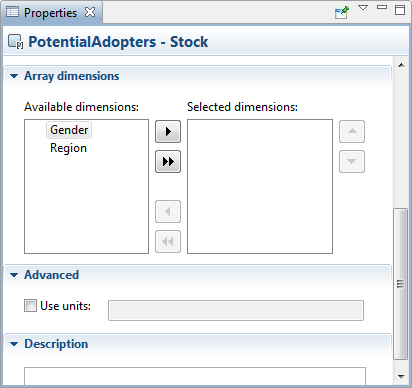5. Define the dimensions of your array variable. Add the dimensions you need from the Available dimensions list to the Selected dimensions list. To add some dimension, select it in the Available dimensions list and click the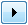button. To add all available dimensions, click the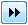button.
6. If needed, you can remove some selected dimensions any time you like. To remove a dimension, select it in the Selected dimensions list and click the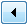button. To remove all selected dimensions, click the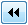button.
7. The order of dimensions is also important. You can reorder the dimensions by clicking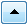and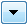buttons.

## Initializing arrays

Some system dynamics variables of type array (stock variables, parameters, constant flow, and dynamic variables) need to be initialized.

AnyLogic provides easy and flexible tool for defining initial values of array elements. This is done because initialization of multidimensional arrays is non-trivial task, first of all as there is no way of intuitive visual representation of multidimensional data.

As arrays can have unlimited number of dimensions, each one possibly containing a great amount of elements, the sole convenient way of defining initial values of an array is layering this array (i.e. partitioning this array to layers) and successive initialization of each resulting layer.

You define a layer by fixing values in all but two dimensions of an array. When we say fix we mean that you select one particular element in the dimension. Thereby each fixation narrows the list of array dimensions by one dimension. Having finished narrowing dimensions to two ones, you get an ability to represent data of the resulting two-dimensional layer in tabular form. To define initial values for all array elements you need to go over all combinations of elements of non-fixed dimensions.

To open the editor of array initial values

1. Select the array in a graphical editor or in the Projects view.
2. Click the Edit... button in the Properties view.
3. The editor will be opened. The editor consists of two panels. In the left panel you define a layer, whose elements you want to initialize. The right panel contains a table where you, in fact, define values of elements of the selected array’s layer.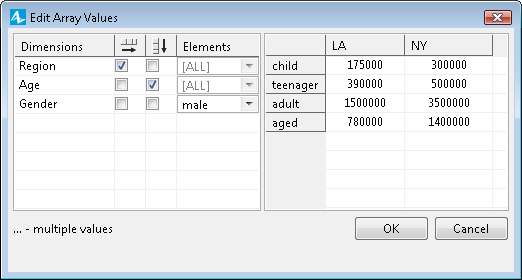To make clear how to initialize array elements, let us illustrate it by a pair of simple examples.

### Initializing all array elements with one value

To initialize all array elements with one value

1. Open the editor of array values.
2. Make sure that in the left table the value [ALL] is selected in all cells of the Elements column.
3. Remove marks from either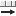and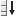columns of this table.
4. In the right table, enter the value you want to assign to all elements of this array.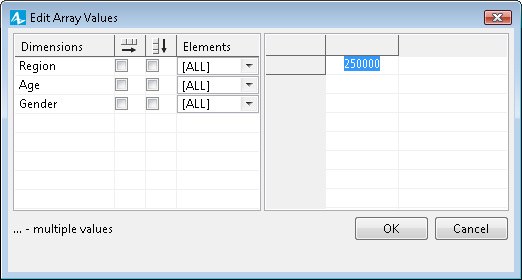5. Click OK when finished.

### Initializing array elements with different values

Assume you are creating a model studying population dynamics in LA and NY. Let’s conditionally categorize people by three characteristics: gender, age and region. These characteristics are comfortably described using dimensions — enumerations: Gender(male, female), Age(child, teenager, adult, aged) and Region(LA, NY).

Let’s study how to initialize three-dimensional array Population[Region, Age, Gender] using our editor of array values:

To initialize all array elements with one value

1. Open the editor of array values.
2. You will see that two dimensions have marks in the left table of the editor: one in thecolumn and one in thecolumn. Marking this or that dimension you can choose dimensions, whose elements you will define in the right table. Elements of the dimension, selected in thecolumn, will be displayed there as rows, while elements of the dimension selected in thecolumn will be displayed as columns: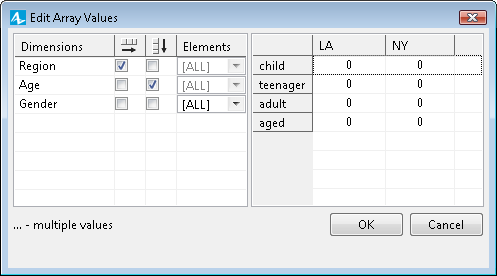3. If you will start entering values now, they will be assigned to the corresponding elements of all non-fixed dimensions of the array, i.e. if you enter, say, 1000000 in the cell adult - LA, thereby you will initialize both number of adult men and adult women in LA with this value.
4. If you want to define different initial values for different elements of Gender dimension, you should fix this dimension as we mentioned above.
5. Let’s first initialize the number of men. Select male in the cell Gender — Elements in the left table. Now you can define the number of men in your model in the right table — each cell of the table defines the value of the corresponding element of the population array, e.g. in the cell aged - NY the number of aged men living in NY can be defined. Define values of all elements of this array’s layer [Region, Age, male]: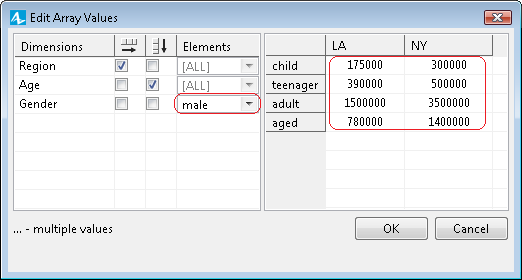6. Now we should define the number of women in the similar way. This time you should select another element of the fixed dimension, whose elements are not fully initialized yet. Choose female in the cell Gender - Elements and define the number of women of each category in the right table: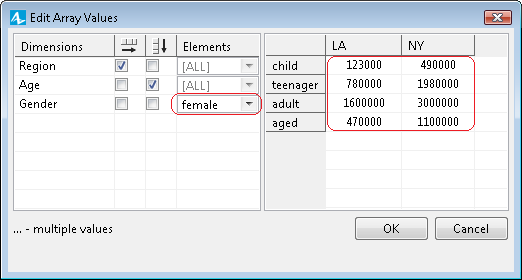7. Now we have finished initialization of array’s layer [Region, Age, female] and therefore the initialization of the whole array (as in this simplest case our array has only two layers since it is three-dimensional and the only dimension we are fixing has only two elements).
8. Click OK to finish the initialization.

You can check whether you have defined initial values correctly by inspecting variable value at the model runtime: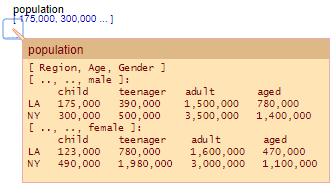The resulting array’s initialization string is displayed in the text form in the Initial value field (or Default value, in the case of a parameter-array). If you need to initialize some another array with similar values, you can simply copy this string to the analogous property of that array and then modify the required values using the editor of array values.

## Defining equations for array variables

There is a number of ways you can define the equation (or equations) for an array stock, flow or dynamic variable.

### Defining the same equation for all array elements

In the simplest case when the equation is the same for all elements of the array, you need only one equation field, where you type the expression, possibly referencing the dimensions of the array variable. For example, consider the stock Population which is an array with the following dimensions:

Region = {NORTH, SOUTH} and Gender = { MALE, FEMALE }

In this case, the equation will look like:

d(Population[Gender, Region])/dt = Births[Gender, Region]-Deaths[Gender, Region]

To set the same equation for all array elements

1. Select the array variable in the graphical editor and go to the Properties view.
2. By default the stock’s formula is generated automatically according to flows flowing and out of this stock, it is so called classic equation mode, for more details please refer to the Classic and custom modes section. The value of inflows i.e. flows that increase stock value, are added and the value of outflows, i.e. flows that decrease the stock are subtracted from the current value of the stock. In the figure below you can see the auto-generated equation formed by AnyLogic for the stock Population from our example, see the disabled field d(Population [Region,Gender])/dt=.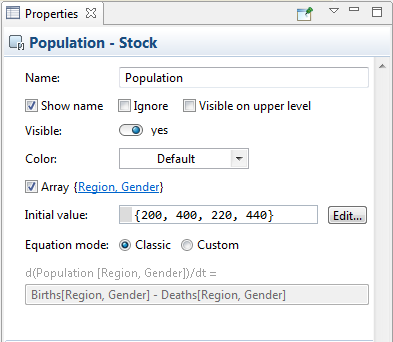3. If you need to overcome the limitations of classic system dynamics formula of the stock anyway and want to edit it by yourself, select the Custom option from the Equation mode group of buttons and specify the formula in the d(stock_name)/dt field.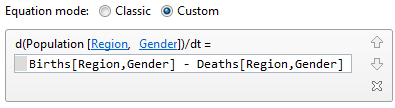4. If in our example Births are the same for MALE and FEMALE and differ only by region (so that variable Births is the array with one dimension Region), you may write: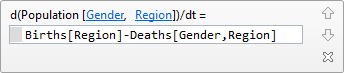The equation above is mapped to a very simple loop construct that looks like this:

for( r : Region )
for( g : Gender )
Population[ r, g ] = Births[ r ] - Deaths[ r, g ]

If birth rate is the same even in different regions, you can use a scalar variable Births in the same equation: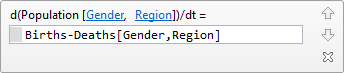### Defining different equations for different element sets of an array

There are cases however when equations are different for different elements of the array or different element sets. Suppose in the population example above people do intensively migrate out of NORTH region, but migration out of SOUTH region is negligible. Then there will be two equations for the stock Population:

d(Population[Gender, SOUTH])/dt = Births[SOUTH] - Deaths[Gender, SOUTH]

d(Population[Gender, NORTH])/dt = Births[NORTH] - Deaths[Gender, NORTH] - OutMigration

To define several equations for different sub-arrays of an array variable

1. Select the variable in a graphical editor or in the Projects view.
2. Go to the Properties view.
3. Specify the list of subscripts identifying element(s) of the array that should get the value calculated by the equation you specify. By default, all array dimensions are selected. You should modify this dimension list by specifying some particular elements for those enumerations that are presented in the sub-array by a subdimension or (in case of enumerations) an individual element. For such dimensions, click on the dimension name in the {...} to the right of the Array check box and choose the subdimension or individual element from the drop-down list.
4. To define the first formula of the example described above, you should do the following: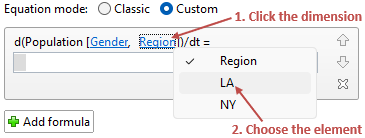5. Enter the formula calculating the initial value for the defined sub-array in the d(<array_name>)/dt= edit box: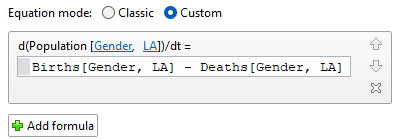6. If you need to define one more formula initializing another sub-array, click the Add formula button and define new formula in the same way.
7. For the described example, you need to define the second equation describing the population of north region habitants: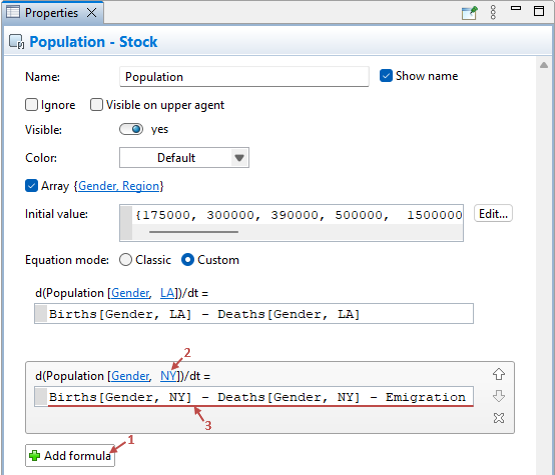8. If needed, you can remove formulas any time you like. To remove a formula, click the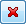button to the right of the formula.

In the equation sets like above you can also refer to element subsets by using subdimensions. For example, if there is dimension Income = { POOR, MIDDLECLASS, WEALTHY } and its subdimension AllButPoor = { MIDDLECLASS, WEALTHY }, you can write for a three-dimensional array Population:

``````d( Population[ Region, Gender, POOR ] )/dt =
Births[ Region ] - Deaths[ Region, Gender, POOR ] - OutMigration

d( Population[ Region, Gender, AllButPoor ] )/dt =
Births[ Region ] - Deaths[ Region, Gender, AllButPoor ]``````

### Referring to other array elements

In some cases you need to refer to a different index of an array in the equation. Suppose you are modeling an ageing chain and your stock Population is an array with one dimension Age = { 0 .. 99 }. The inflow for an element of the array is the outflow of the element with the previous index for all element but 0, and for the element 0 the inflow is Births. To implement this you may define two subdimensions of Age: Age0 = { 0 } and AgesAllBut0 = { 1 .. 99 } and write:

``````d( Population[ Age0 ] )/dt =
Births - Deaths[ Age0 ] - Ageing[ Age0 ]

d( Population[ AgesAllBut0 ] )/dt =
Ageing[ AgesAllBut0 - 1 ] - Deaths[ AgesAllBut0 ] - Ageing[ AgesAllBut0 ]``````

To understand how it works it makes sense to map this into loop construct:

``````for( a : 0 }
Population[ a ] = Births - Deaths[ a ] - Ageing[ a ]

for( a : 1..99 }
Population[ a ] = Ageing[ a-1 ] - Deaths[ a ] - Ageing[ a ]``````

As you can see, the dimension names are simply substituted with loop indices in the equations. This gives you a high degree of flexibility when you define equations. You can define the dependency of element with a certain index on the element of same of another array having arbitrary other index.

## Array API

AnyLogic supports a set of functions you can use to perform operations over arrays.

Aggregation functions

Sometimes you need to perform aggregation operations on elements of arrays. Aggregation functions over arrays are listed in the table below.

Function Description
double average() The mean value of the aggregated elements: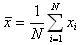boolean hasNegativeValues() Checks if there are any negative elements in the hyper array. Returns true if at least one element is negative, otherwise false.
double max() Maximum value of the aggregated elements.
double min() Minimum value of the aggregated elements.
double prod() The product of the elements: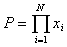int size() Returns the total number of elements in the hyper array.
double stddev() Standard deviation: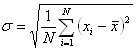double sum() The sum of the aggregated elements: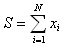Array functions for changing elements' values

The following set of functions allows you to manage the values of elements in a hyper array. You can use indices to define to which elements a value should be added or subtracted from and the parameters to define how much exactly.

int INDEX_CAN_VARY is a special constant that, being placed at an index position, tells the methods that perform operation over subsets of hyper array elements that they can vary the corresponding index. Other indices are fixed. INDEX_CAN_VARY can be used to increment, to decrement, or to multiply across all the elements of a particular dimension.

Function Description
void increment(int... indexes) Increments element(s) with the given index(es) by 1.

indexes — the array of indices, INDEX_CAN_VARY for varying ones
void incrementBy(double value, int... indexes) Increments element(s) with the given index(es) by the given value amount.

value — the value to add to and to subtract from array elements
indexes — the array of indexes, INDEX_CAN_VARY for varying ones
void decrement(int... indexes) Decrements element(s) with the given index(es) by 1.

indexes — the array of indexes, INDEX_CAN_VARY for varying ones
void decrementBy(double value, int... indexes) Decrements element(s) with the given index(es) by the given -value amount.

value — the value to add to and to subtract from array elements
indexes — the array of indexes, INDEX_CAN_VARY for varying ones
void multiply(double factor, int... indexes) Multiplies element(s) with the given index(es) by the given factor.

factor — the factor to apply to array elements

You can perform calculations on a subset of an array. In this case you should enumerate the dimensions forming the sub-array as function arguments. For example, if you have an array people with dimensions ( Region, Gender, AgeGroup ), calling: